# The Significance of Burley’s And Csapo’s Characteristic Equation And Its Root Solution

Peter Burley and Laszlo Csapo:

Our references in this section are [Burley, 1992-2] and [Burley and Csapo, 1992-1].

Burley, Peter and Csapo, Laszlo, (1992) Money Information in Lonergan-von Neumann Systems, Economic Systems Research, Vol 4, No. 2, 1992 [Burley and Csapo, 1992-1]

Burley, Peter (1992) Evolutionary von Neumann Models, Journal of Evolutionary Economics 2 , 269-80 [Burley, 1992-2]

We consider a game-theoretic, von Neumann model of the transitional process from an initial stationary state to a more abundant stationary state, with matrix A of inputs and matrix B of outputs containing explanatory functional variables.

This treatment demonstrates the principles and laws of Functional Macroeconomic Dynamics.  The reader must ”go to your sources” for details, many of which will not be parroted here.  Pencil-to-paper study of both models will generate understanding of the immanent intelligibility of the dynamic economic process. The explanatory functional flows will be purely relative to one another, purely functional, purely dynamic, and, by shortened intervals, supply-demand concomitant.  The theory of Functional Macroeconomic Dynamics is an explanatory field theory comprised totally of endogenous interdependencies; the theory is prior to, and more fundamental than, a theory based on a psychology of utility and preference.

The key ideas and conclusions of this section may be stated here and now:

• The generalized eigenvalue problem relating input-matrix A and output-matrix B is given by the characteristic equation, which gathers both ends of the process to yield a mechanical structure or a pattern of laws.  This characteristic equation demonstrates in one abstract formalism that all of the interdependent, explanatory functional flowings of products and money are unitary. The theory interrelates and implicitly defines these endogenous, explanatory flows

B – (α = β)A = 0  the characteristic equation

• The characteristic equation’s root solution gives the growth factor α and the rate of return β.  The growth factor α should normatively equal the interest rate factor β.
• Thus, the characteristic equation reveals a normativity and a normative theory
• By its root solution, the characteristic equation contains the immanent normative growth factor and interest factor which reveal the velocity and accelerator rhythms and enables the determination of immanent normative relative production intensities and normative relative product pricings applicable to the possible secondary coincidental factors of money supply, labor supply, and state of technology in any and every instance.
• By means of the strategy vectors for productive intensities and relative pricings, the characteristic equation virtually contains the mutual constraints among functionings for productive and financial equilibrium in both stable and expansionary phases of the process.
• The characteristic equation demonstrates that the interest rate is a complicated internal relation among many flows, not the simplistic external lever or magic wand of the Fed and the textbooks.
• The Macroeconomic Interest Rate is (β-1); it is one abstract primary relativity among a coherent set of fundamental and superstructural abstract primary relativities, which all-together explain the objective economic process in any and every instance, no matter the coincidental secondary determinations from the non-systematic manifold.
• The Macroeconomic Interest Rate can be understood as an endogenous rate of return; its endogenous change can be clearly explained and is not a mysterious external shock.
• This interest rate is not to be conceived and mistakenly used as a magical external lever; its manipulation may prove to be counterproductive having unintended consequences.
• The Macroeconomic Interest Rate is, thus, one concept among several concepts tumbling out from an insight relating, at once, the aggregate current, interdependent, mutually-conditioning, explanatory flows which constitute, and are explanatory of, the objective process.
• These functional flows implicitly define one another by the functional relations in which they stand with one another in the constitution of the current, dynamic process. In the characteristic-equation formalism. the terms (velocitous flows) define the relations and the relations define the terms.
• By applying scaling factors of total labor to the primal (production) side and money supply to the dual side, one gets a measurement of GDP or GDFF.

(The task of the macroeconomist) is to work out the correlations that exist between the velocity and accelerator rhythms of production and the corresponding rhythms of income and expenditure.  The set of such correlations constitutes the mechanical structure, a pattern of laws that stand to economic activity as the laws of mechanics to buildings and machines. [CWL 21, 43]

The characteristic equation of the inputs and outputs of the models is an implicit equation; it demonstrates a) that the concrete process is explained by the relations of the abstract primary relativities of constituent functionings among themselves, applied to secondary concrete determinations of the actual prices, quantities, and technology of a non-systematic manifold, and b) that the primary relativities are expressed as implicit equations constituting the implicit definitions of interdependent functional flows by the functional relations in which they stand with one another.

We first refer to Burley’s introductory simplified two-by-two input and output matrices in the format of linear algebra. [Burley 1992-2, 275]  We ask the reader to visit [Burley 1992-2] for the contents and the context of the symbols and for better renditions of the matrices.

αA = B;   B – αA = 0

with relative intensity vectors x, and relative pricing vectors y

αxAy = xBy

and with consumption units C, stock of capital K, labor L = l + l’; consumption units output quantity k, rate of depreciation d,

A                           B

C         K                C           K

¦cl         1 ¦           ¦k          1-d¦

¦cl’        0 ¦          ¦ 0         1¦

Next we print the characteristic equation of these matrices. The growth rate and the equal interest rate will be -1) and -1) respectively; α is the root of the primal side of the characteristic equation.  The consumption units c are c = k/(l+dl’); the growth factor a will, thus, equal 1 and the interest factor β will equal 1;

k-αcl              1-d-α1

= 0

αcl’                1-α0

or, with the characteristic equation’s growth factor α= 1, the characteristic equation is expressed as

k – cl + d + cl’ = 0

Note that the characteristic equation is an implicit equation and the terms implicitly define and mutually condition one another.  The variables must be assigned magnitudes, but the magnitudes will be consistent with one another within the constraints implicit in the primary relativity.  The analysis is purely relative. Terms are defined by the relations in which they stand with one another.  In order for this characteristic equation, representing aggregates of activities, to equal zero, any variation in one term requires a compensating change in another term.  The terms are mutually defining, mutually conditioning, mutually supporting, and mutually constraining.

The Game-Theory normalized strategy for the (relative production intensities vector) player can then be derived: x = [1/(1+d), d/(1+d)] and for the (relative price intensities vector) player: y = [(l+dl’)/(l+dl’+kl’), kl’/(l+dl’+kl’)]

Note also that the strategy intensities are mutually conditioning and mutually constraining. An excess of one intensity implies waste in its output and insufficiency in another output.  There are systematic constraints.

This general formalism may be expanded to larger matrices so as to include more detail, but the general form continues to be generally applicable and universally relevant; it is grounded in the fully explanatory relations of quantities among themselves; it is purely relative and does not change with changes in the secondary determinations of the scaling factors: labor supply L, money supply Y, and technology multiplier k; it is an abstract primary relativity which may be applied to these secondary determinations in any instance. The mathematical meaning of Burley’s pattern is general; it can accommodate any substitutions of labor supply, money supply, or productivity multiplier.

…  The mathematical meaning of an expression resides in the distinction between constants and variables and in the sign or collocations that dictate operations of combining, multiplying, summing, differentiating, integrating, and so forth. It follows that, as long as the symbolic pattern of a mathematical expression is unchanged, it’s mathematical meaning is unchanged.  Further, it follows that if a symbolic pattern is unchanged by any substitutions of a determinate group, then the mathematical meaning of the pattern is independent of the meaning of the substitutions. [CWL 3, 18-19/43]

Next we show Burley’s slightly more complicated characteristic equation for three-by-three inputs and outputs and the Newton-Raphson root solution for this three-by-three transitional expansionary process. [Burley, 1992, 277]

k  – βlc                         0                      l(1 – β)

kN βlNc                    1- dNβ            lN(1 – β)              = 0

βl’Nc                          1                      l’N(1 – β)

This characteristic equation has two root-solutions: growth factors α and interest factors β

a1 (= β1) =1, and

α2 (= β2) = 1+(lkN-lNk)/l’Nk)-dN.

from which the strategies for relative intensity and relative pricing may be derived.

The point is that, while Burley’s evolutionary model is a very-much simplified linear model, its characteristic equation demonstrates clearly the primary relativity – independent of human psychology; and general and universally relevant in any instance –  axAy = xBy, or xByaxAy = 0, which can be applied to the secondary determinations of money supply, labor supply, and  technology in the non-systematic manifold.  All the primary-relational terms are interdependent; they implicitly define one another; they mutually condition one another.

By shortening the time interval between inputs and outputs, the inputs and outputs can become practically concomitant, with any slight delays or lags supportable by the temporary credit function. Thus we always deal with the “current process”;  and the growth rate α-1 and interest rate β-1 are purely-current relativities whose maturity is the instantaneous now.

The productive process is, then, the (current) aggregate of activities proceeding from the potentialities of nature and terminating in a standard of living.  Always it is the current process, and so it is distinguished both from the natural resources, which it presupposes, and from the durable effects of past production. [CWL 15, 20]

Regarding concomitance, and with a sidelong glance at the familiar Diagram of Rates of Flow,

A condition of circuit acceleration was seen in section 15 to include the keeping in step of basic outlay, basic income, and basic expenditure, and on the other hand, the keeping in step of surplus outlay, surplus income, and surplus expenditure.  Any of these rates may begin to vary independently of the others, and adjustment of the others may lag.  But any systematic divergence brings automatic correctives to work.  The concomitance of outlay and expenditure follows from the interaction of supply and demand.  The concomitance of income with outlay and expenditure is identical with the adjustment of the rate of saving to the requirements of the productive process. [CWL 15, 144]

Thus the process is not explained, as it is in the DSGE methodology, primarily as a set of discontinuous, fitful shocks and sequential adjustments but rather primarily as a practically-continuous set of current interrelated functional flows, concomitantly interdependent, mutually-defining, mutually-conditioning, mutually supporting, and mutually constraining one another.  In both Lonergan’s Pure Cycle of Gross Domestic Functional Flows and in Burley and Capo’s linear, game-theoretic, matrix  models, the economic process is explained by equations as a current and continuous complex system of implicitly-defined velocitous flows.  Attention is focused on second-order differential equations of acceleration represented by factors of increase α and β.

The root-solution Macroeconomic Interest Rate is not the Federal Reserve Bank’s or DSGE’s magical external lever; and both the interest rate at the heart of the IS-LM model and the pricing at the heart of the AD-AS model  are to be understood, not as momentary external givens, but rather in the light of these significant explanatory flows.

Lonergan agreed with Schumpeter on the importance of systematic or analytic framework in order to explain, rather than merely record or describe, the aggregate phenomena of macroeconomics; … and he agreed that the economist had to know what are the significant variables in the light of which price changes are to be interpreted. According to Lonergan, standard economic theory had successfully achieved none of these desiderata. [CWL 15, Editors’ Introduction liii]

In a theoretically stable economy, merely reproducing itself in a constant velocity period after period, the velocities of flows would be indicated by α = β= 1.  Thus McShane understands and insists that the creative key context in the macroeconomics of a creatively expanding economy is the shift of focus to differential equations representing accelerative growth.

Taking into account past and (expected) future values does not constitute the creative key transition to Functional Macroeconomic Dynamics.  Those familiar with elementary statics and dynamics will appreciate the shift in thinking involved in passing from (static) equilibrium analysis … to an analysis where attention is focused on second-order differential equations, on d2θ/dt2, d2x/dt2, d2y/dt2, on the primary relativities of a range of related forces, central, friction, whatever.  Particular secondary boundary conditions in Functional Macroeconomic Dynamics, past and future pricings and quantities, are relatively insignificant for the analysis of the primary relativity immanent in, and applicable to, every instance of the process.  What is significant is the Leibnitz-Newtonian shift of context. [partial paraphrase of McShane, 1980, 127]

Models demonstrate fundamental principles and laws.  Macroeconomic models demonstrate the primary relativities which govern the aggregative process. They bring to light the “formal cause” of the process. Lonergan provides a model of the Pure Cycle based upon foundational, precise analytical distinctions in the structure of the productive process. Other formalisms may be used to explain the process:

• Burley’s game-theoretic, linear axAy = xBy is another explanatory model of Lonergan’s Gross Domestic Functional Flows.
• One could also construct a logistic growth model of the process, centered on Stewart’s [Stewart, 2008, 568, and 592-95]

dP/dt = kP(1 – P/K)     or, alternatively    dP/dt = kP(K – P)

with solution:  P(t) = K/(1+Ae-kt) where A = (K-P0)/P0

or Strang’s  [Strang, 1991, 261 ff.]

dy/dt = cy – by2.

GDFF = P’Q’ + ΠΚ= p’a’Q’basic circuit costs+ p”a”Q” surplus circuit costs+ π”α”Κ”expansionary outlays+ π”α”Κ”R&M outlays

Finally, we append an extensive Table of Results for Burley’s Transitional Model in the Appendix to (Burley, 1992-2, 279) as follows:(Note to self and reader: research erratum of parenthesis in denominator)

A sampler from (Burley and Csapo, 1992-1) and (Burley, 1992-2):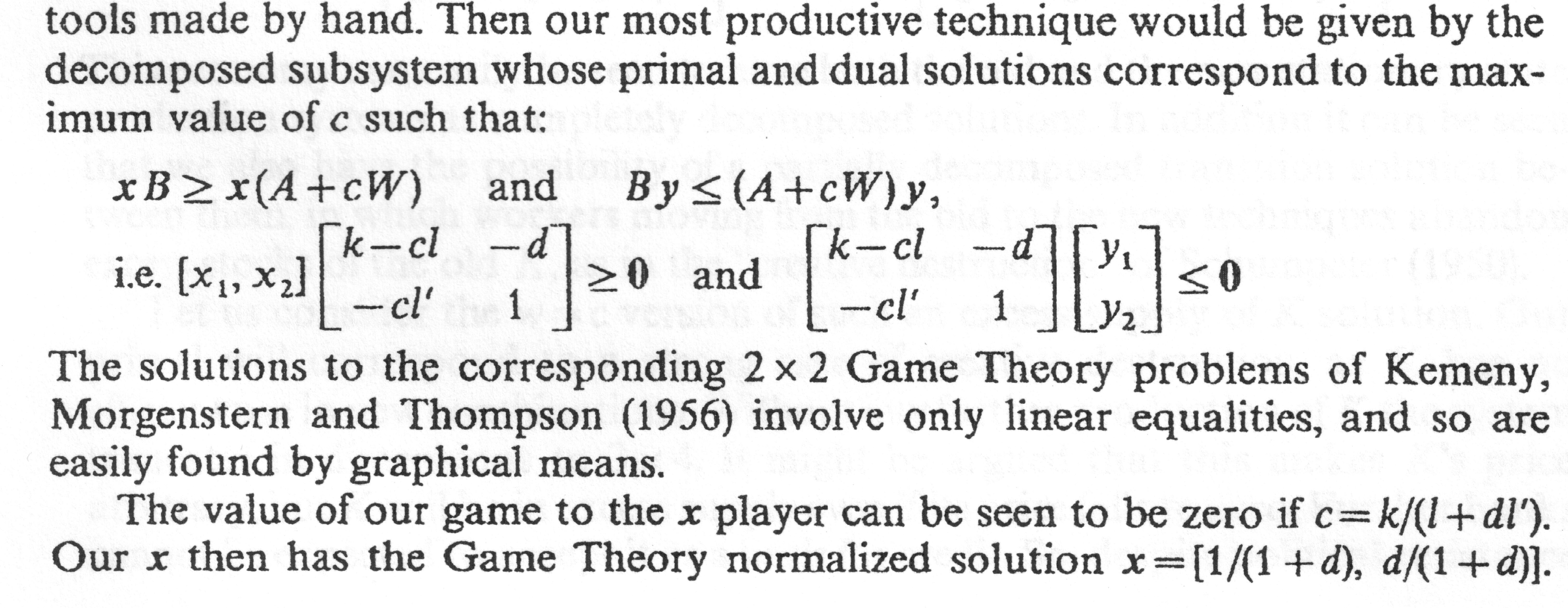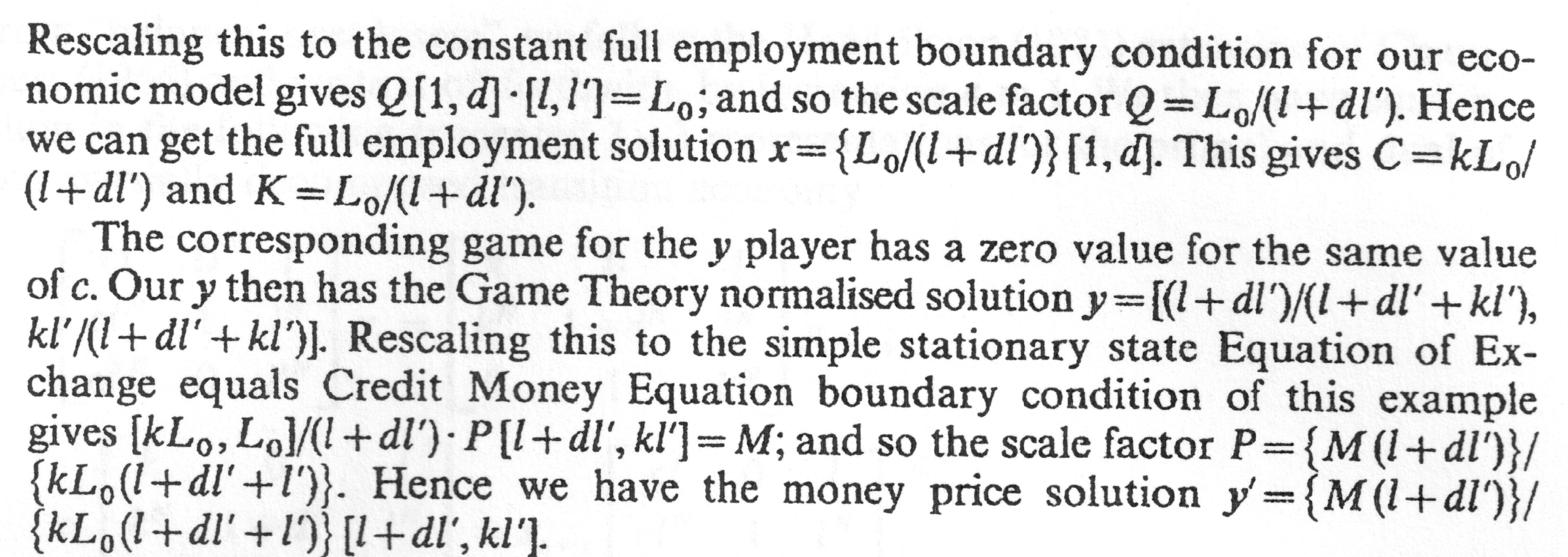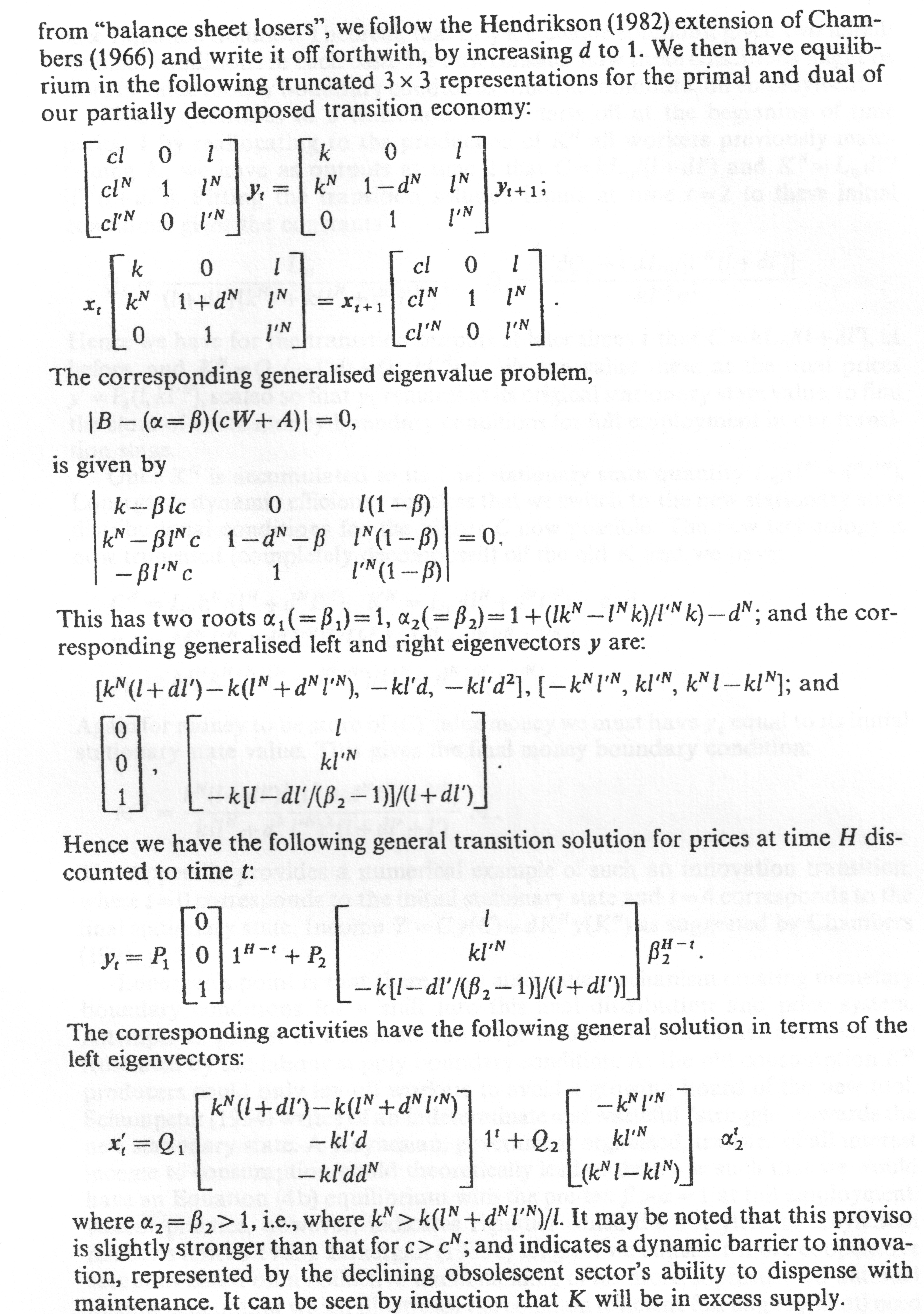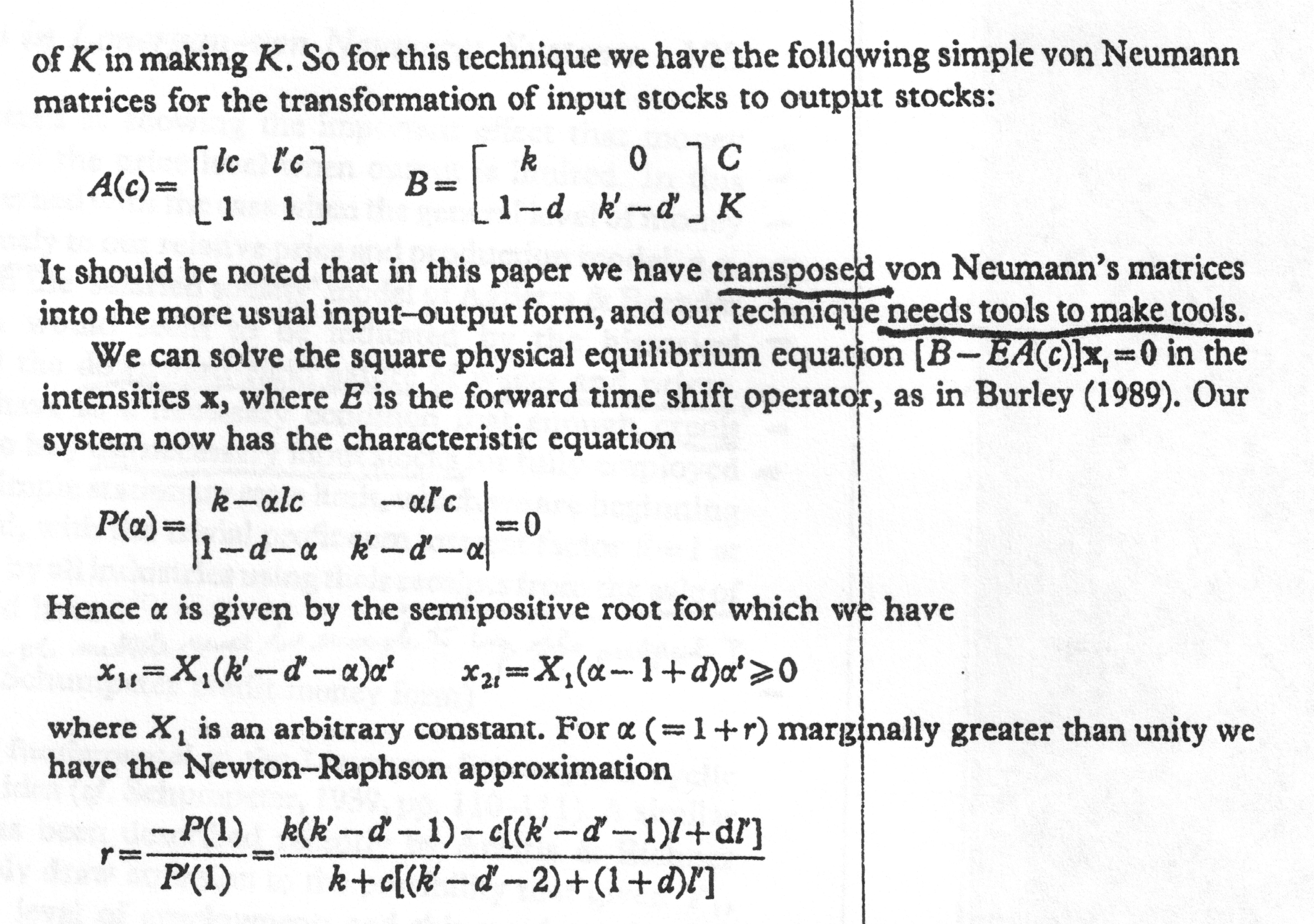Burley + Csapo 2002-1, 134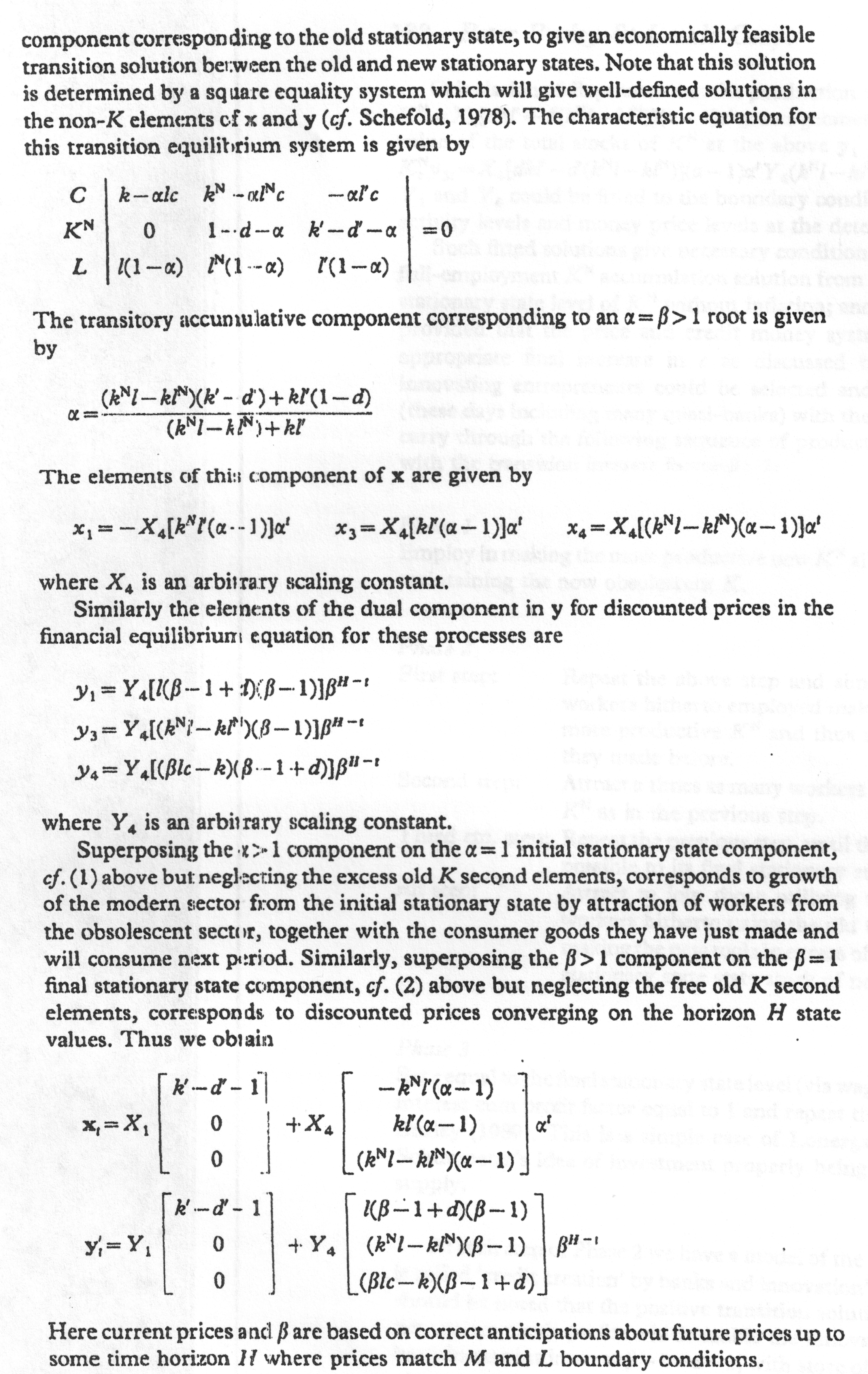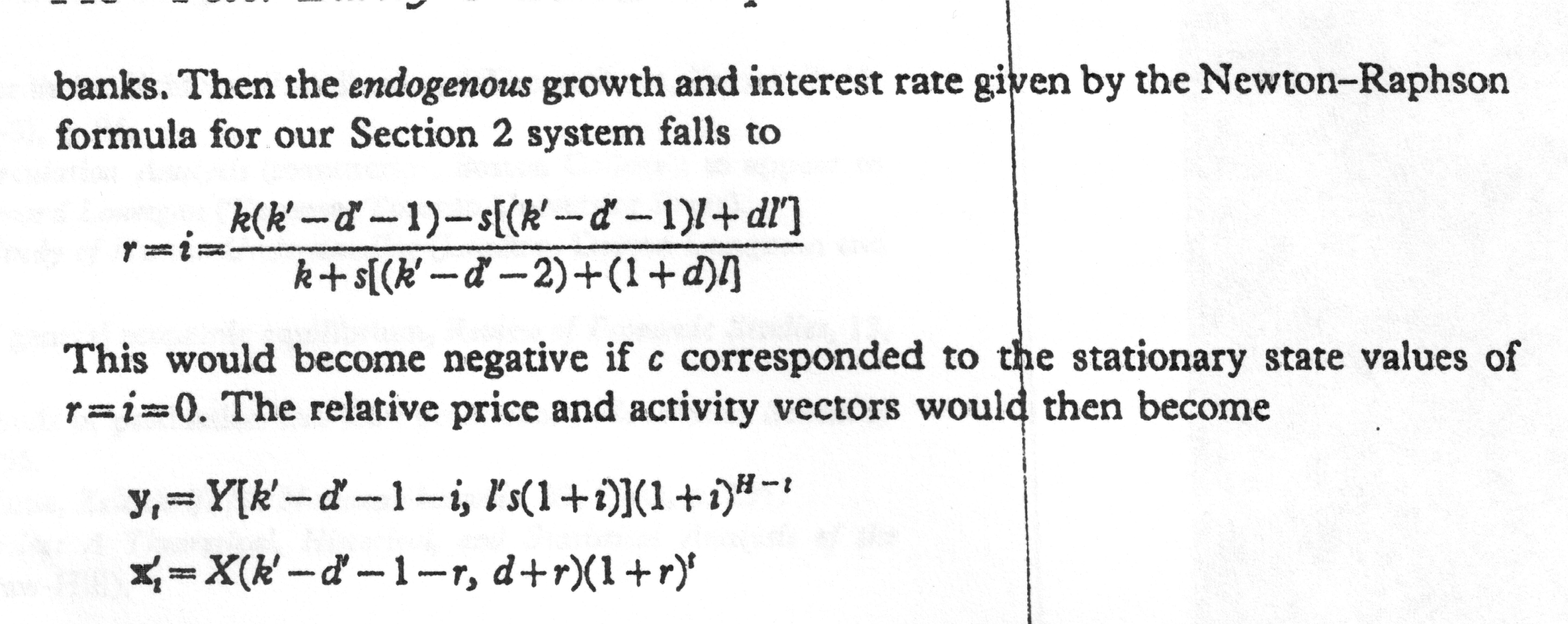xxx